您可以捐助，支持我们的公益事业。 1元 10元 50元 认证码：必填求知 文章 文库 Lib 视频 iPerson 课程 认证 咨询 工具 讲座 Modeler Code要资料订阅捐助

 256 次浏览 评价： 好 中 差
2020-8-12

 编辑推荐: 这篇文章中，我们挑选了24个用于数据科学的Python库。这些库有着不同的数据科学功能，例如数据收集，数据清理，数据探索，建模等，接下来我们会分类介绍。 本文来自于 微信公众号 - 磐创AI（xunixs），由火龙果软件Alice编辑，推荐。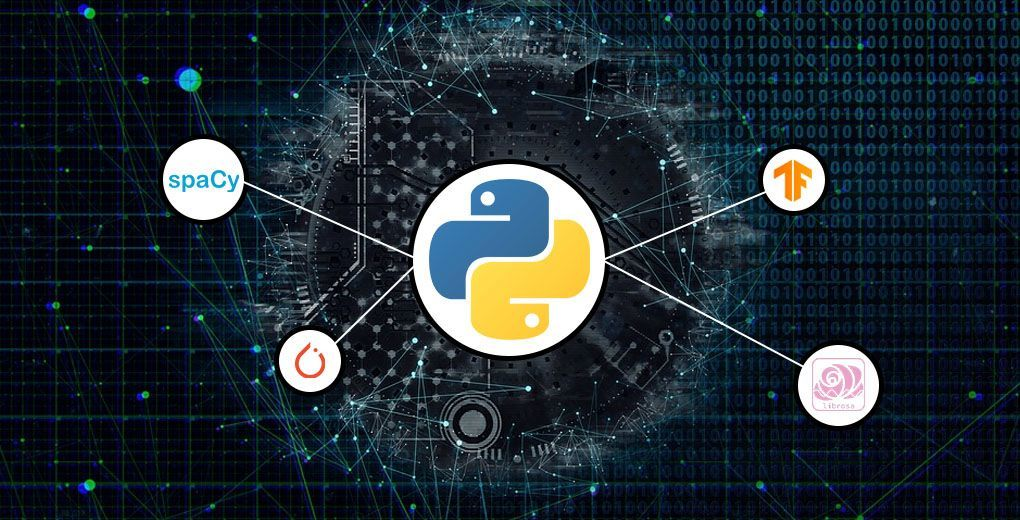Beautiful Soup

Scrapy

Selenium

Pandas

PyOD

NumPy

Spacy

Matplotlib

Seaborn

Bokeh

Scikit-learn

TensorFlow

PyTorch

Lime

H2O

Librosa

pyAudioAnalysis

OpenCV-Python

Scikit-image

Pillow

Psycopg

SQLAlchemy

/* Beautiful Soup */

Beautiful Soup是一个HTML和XML解析器，它为解析的页面创建解析树，用于从网页中提取数据。从网页中提取数据的过程称为网络抓取。

 pip install beautifulsoup4

 #!/usr/bin/python3 # Anchor extraction from html document from bs4 import BeautifulSoup from urllib.request import urlopen with urlopen('LINK') as response: soup = BeautifulSoup (response, 'html.parser') for anchor in soup.find_all('a'): print(anchor.get('href', '/'))

/* Scrapy */

Scrapy是另一个用于Web抓取的超级有用的Python库。它是一个开源和协作框架，用于从网站中提取您需要的数据。它使用起来快速而简单。

pip install scrapyimport scrapy class Spider(scrapy.Spider): name = 'NAME' start_urls = ['LINK'] def parse(self, response): for title in response.css('.post-header>h2'): yield {'title': title.css('a ::text').get()} for next_page in response.css('a.next-posts-link'): yield response.follow(next_page, self.parse)

/* Selenium */

Selenium是一种用于自动化浏览器的流行工具。它主要用于行业测试，但对于网络抓取也非常方便。实际上，Selenium在IT领域变得非常受欢迎，所以我相信很多人至少会听说过它。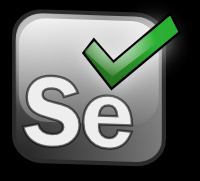/* Pandas */

Pandas需要预先安装Python或Anaconda，这里是需要的代码：

 pip install pandas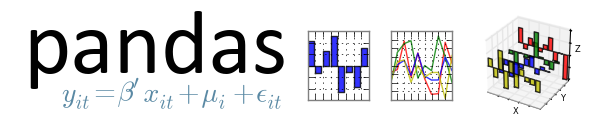Pandas提供的功能如下：

DataFrame对象操纵数据等等！

12用于数据操作的Python中有用的熊猫技术

CheatSheet：使用Python中的Pandas 进行数据探索

/* PyOD */

PyOD是一个全面且可扩展的Python工具包，用于检测外围对象。异常检测基本上是识别与大多数数据显着不同的稀有项目或观察。

 pip install pyod

/* NumPy */

NumPy是一个开源库，有多个贡献者。它预先安装了Anaconda和Python，这里是安装它的代码：

 pip install numpy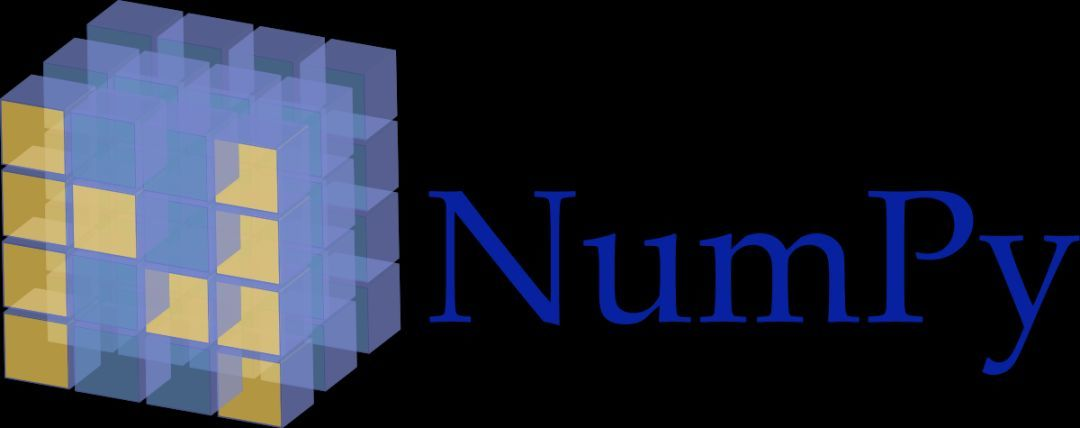# 创建数组 import numpy as np x = np.array([1, 2, 3]) print(x) y = np.arange(10) print(y) # output - [1 2 3] # [0 1 2 3 4 5 6 7 8 9] # 基本操作 a = np.array([1, 2, 3, 6]) b = np.linspace(0, 2, 4) c = a - b print(c) print(a**2) #output - [1. 1.33333333 1.66666667 4. ] # [ 1 4 9 36]

/* SpaCy */

spaCy是一个超级有用且灵活的自然语言处理（NLP）库和框架，用于清理文本文档以进行模型创建。与用于类似任务的其他库相比，SpaCy更快。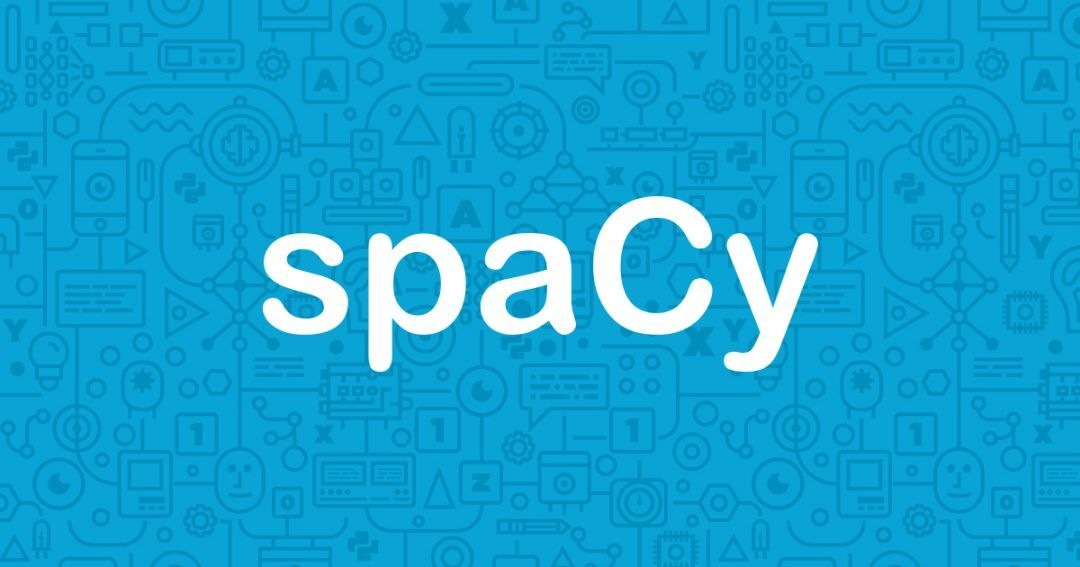/* Matplotlib */

Matplotlib是Python中最流行的数据可视化库。它允许我们生成和构建各种图表。它可以与Seaborn一起使用。

 pip install matplotlib

pip install matplotlib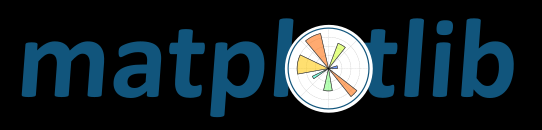# 直方图 %matplotlib inline import matplotlib.pyplot as plt from numpy.random import normal x = normal(size=100) plt.hist(x, bins=20) plt.show()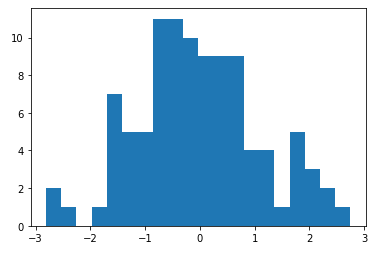# 3D图 from matplotlib import cm from mpl_toolkits.mplot3d import Axes3D import matplotlib.pyplot as plt import numpy as np fig = plt.figure() ax = fig.gca(projection='3d') X = np.arange(-10, 10, 0.1) Y = np.arange(-10, 10, 0.1) X, Y = np.meshgrid(X, Y) R = np.sqrt(X**2 + Y**2) Z = np.sin(R) surf = ax.plot_surface (X, Y, Z, rstride=1, cstride=1, cmap=cm.coolwarm) plt.show()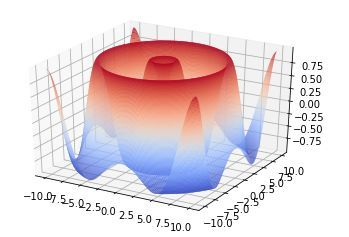/* Seaborn */

Seaborn是另一个基于matplotlib的绘图库。它是一个python库，提供高级界面来绘制有吸引力的图形。matplotlib可以做什么，Seaborn只是以更具视觉吸引力的方式做到这一点。

Seaborn的一些功能是：

 pip install seaborn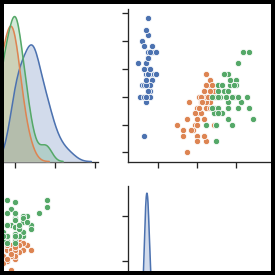import seaborn as sns sns.set() tips = sns.load_dataset("tips") sns.relplot(x="total_bill", y="tip", col="time", hue="smoker", style="smoker", size="size", data=tips);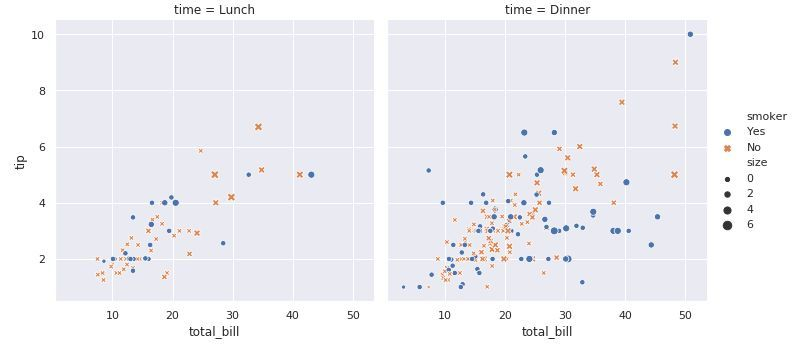/* Bokeh */

Bokeh是一个交互式可视化库，面向现代Web浏览器进行演示。它为大量数据集提供了多种图形的优雅构造。

Bokeh可用于创建交互式图表，仪表板和数据应用程序。安装代码：

 pip install bokeh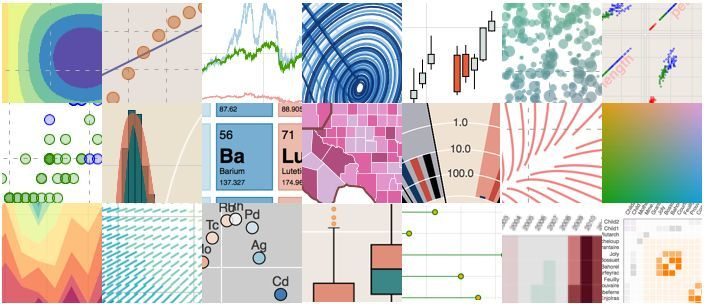256 次浏览     评价： 好 中 差订阅捐助
 相关文章 手机软件测试用例设计实践 手机客户端UI测试分析 iPhone消息推送机制实现与探讨 Android手机开发（一）
 相关文档 Android_UI官方设计教程 手机开发平台介绍 android拍照及上传功能 Android讲义智能手机开发
 相关课程 Android高级移动应用程序 Android系统开发 Android应用开发 手机软件测试
 最新课程计划

 最新文章 简述Matplotlib Python三维绘图--Matplotlib Python数据清洗实践 PyTorch实战指南 Python爬虫与数据可视化 最新课程 Python应用开发最佳实践 Python+数据分析+tensorflow Python 编程方法和应用开发 人工智能+Python＋大数据 Python及数据分析 成功案例 某通信设备企业 Python数据分析与挖掘 某银行 人工智能+Python+大数据 某领先数字地图提供商 Python数据分析与机器学习 北京 Python及数据分析 某金融公司 Python编程方法与实践培训